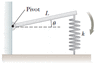# Simple Harmonic Motion and angular frequency

Abid Rizvi

## Homework Statement

A horizontal plank of mass m and length L is pivoted at one end. The plank's other end is supported by a spring of force constant k (see the figure below). The plank is displaced by a small angle θ from its horizontal equilibrium position and released. Find the angular frequency with which the plank moves with simple harmonic motion. (Use any variable or symbol stated above as necessary.)

## Homework Equations

##\omega## = ##\frac{\tau}{I}## (where omega is angular frequency)
F = kx

## The Attempt at a Solution

So I said the force that puts the plank back to equilibrium is kx. Using the definition of arc length, I said kx = k##\theta##L. A force of Mg is also acting on the plank, so I had the total torque = K##\theta##L-Mg*##\frac{L}{2}## I know that the moment of inertia is ##\frac{1}{3}##M##L^2##. Using the formula for ##\omega##, I had ##\sqrt{\frac{k\theta L-Mg\frac{L}{2}}{3ML}}## But this is incorrect. What am I doing wrong?

#### Attachments

•15-p-057.gif
5 KB · Views: 573
Last edited: